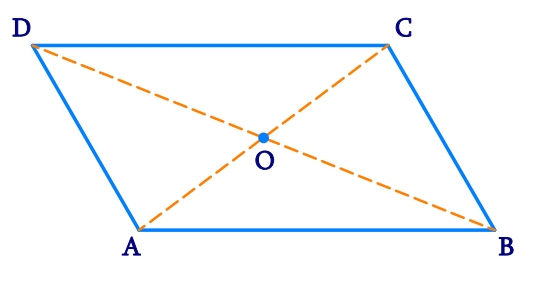# Ex.3.3 Q1 Understanding Quadrilaterals Solution-Ncert Maths Class 8

Go back to  'Ex.3.3'

## Question

Given a parallelogram $$ABCD$$. Complete each statement along with the definition or property used.

(i)$$\, AD\, = \,\_\_\_\_\_\_\_\_\_$$

(ii)$$\, \angle DCB\, = \,\_\_\_\_\_\_$$

(iii)$$\,OC\, = \,\_\_\_\_\_\_\_\_$$

(iv) $$\,m\,\angle DAB\,\!\!+\!\!\,m\,\angle CDA\,\!\!=\_\_\_\_\_\_\_$$Video Solution
Ex 3.3 | Question 1

## Text Solution

What is Known?

$$ABCD$$ is a parallelogram.

What is Unknown?

\begin{align}&{\rm{AD}},\angle DCB,\,{\rm{OC}},\,\,\\&{\rm{m}}\angle DAB +\!{\rm{m}}\angle CDA\end{align}

Reasoning:

We can use the properties of parallelogram to determine the solution.

Steps:

i) The opposite sides of a parallelogram are of equal length.

$${\rm{AD }} = {\rm{ BC}}$$

(ii) In a parallelogram, opposite angles are equal in measure.

$$\angle {\rm{DCB }} = {\rm{ }}\angle {\rm{DAB}}$$

(iii) In a parallelogram, diagonals bisect each other. Hence,

$${\rm{OC }} = {\rm{ OA}}$$

(iv)In a parallelogram, adjacent angles are supplementary to each other. Hence,

$$m\angle {\rm{DAB }} + {\rm{ m}}\angle {\rm{CDA }} = {\rm{18}}0^\circ$$

Learn from the best math teachers and top your exams

• Live one on one classroom and doubt clearing
• Practice worksheets in and after class for conceptual clarity
• Personalized curriculum to keep up with school